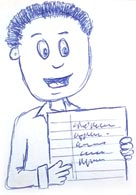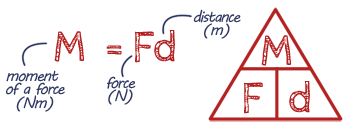# Forces

## Key Words

Vectors - have a magnitude and direction.

Scalar - only have magnitude and no direction.

Contact force - when two objects are touching for a force to act.

Non-contact force - objects do not need to be touching for the force to act.

Interaction pair - a pair of forces that are equal and opposite and act on two interacting objects.

Mass - the amount of 'stuff' in a object.

Weight - the force acting on an object due to gravity.

Gravitational Force - the force of attaction between masses.

Resultant Force - the overall force on a point or object.

Equilibrium - when the forces on an object are balanced.

Moment - the turning effect of a force.

Levers - increase the distance from the pivot at which the force is applied.

Gears - circular discs with 'teeth' that transmit rotational effects.

Also see Motion, Force & Motion, Force & Pressure.

## Revision NotesPrint out revision notes

Need more paper?
Print more

## EQUATION Gravitational Field Strength## EQUATION Force applied to a spring## EQUATION Moment## PRACTICAL Investigating springs

Watch the video and have a go at writing out the steps of the practical.

Note paper for practical